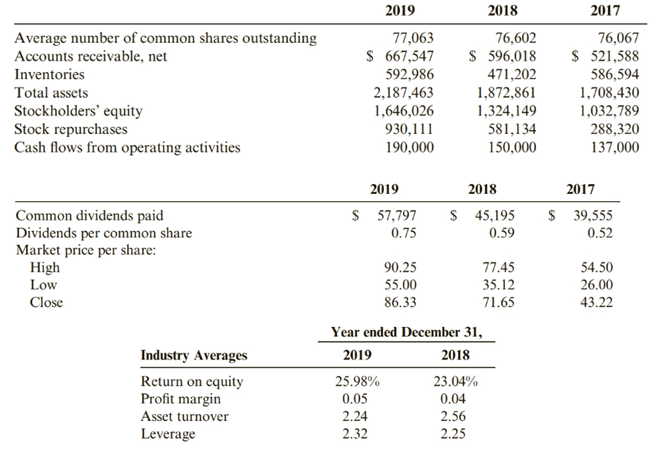Chapter 12, Problem 92PSA### Cornerstones of Financial Accounti...

4th Edition
Jay Rich + 1 other
ISBN: 9781337690881

#### Solutions

Chapter
Section### Cornerstones of Financial Accounti...

4th Edition
Jay Rich + 1 other
ISBN: 9781337690881
Textbook Problem
23 views

# A Preparation of RatiosRefer to the financial statements for Burch Industries in Problem 12-89A and the following data.Required:1. Prepare all the financial ratios for Burch for 2019 and 2018 (using percentage terms where appropriate and rounding all answers to two decimal places).2. CONCEPTUAL CONNECTION Explain whether Burch’s short-term liquidity is adequate.3. CONCEPTUAL CONNECTION Discuss whether Burch uses its assets efficiently.4. CONCEPTUAL CONNECTION Determine whether Burch is profitable.5. CONCEPTUAL CONNECTION Discuss whether long-term creditors should regard Burch as a high-risk or a low-risk firm.6. Perform a Dupont analysis (rounding to two decimal places) for 2018 and 2019.

To determine

(a)

An organization’s liquidity is its capacity to meet its short-term financial commitments. Liquidity proportions endeavor to quantify an organization’s capacity to satisfy its short-term obligation commitments. This is finished by contrasting an organization’s most fluid resources, those that can be effectively changed over to money, with its short-term liabilities.

To discuss:

Calculate all financial ratio.

Explanation

For 2019

Short-term liquidity ratios

1. Current ratio =current Assetscurrent liabilities=1,620,647452,564=3.582. Quick ratio =quick assetscurrent liabilities=985209452,564=2.183. cash ratio = cash + short term investmentcurrent liabilities=291,284452,564=0.644. operting cash flow ratio = cash flow from operating activitycurrent liabilities=190,000452,564=0.42

debt management ratio5. long-term debt to equity ratio=long-term debt total equity=15,0331,542,486=0.016. Debt to equity ratio=Total liabilitiesTotal equity=541,4371,542,486=0.3567. Long-term debt to total assets ratio= long-term debt Total Assets=15,0332,187,463=0.0078. Debt to total assets ratio = Total liabilitiesTotal assets=541,4372,187,463=0.25

Assets Efficiency ratios

9. Accounts Receivable turnover ratio=net credit sales average accounts receivable=3,930,984631,782.5=6.2210. inventory turnover ratio =cost of goods soldaverage inventory turnover=2,386,993532094=4.4811. assets turnover ratio = Net salesaverage total assets=3,930,9842030162=1.93

Profitability ratio

12. Gross Profit Percentage=gross profitNet sale=15439913,930,984=0.4013. Return on assets =Net income +[interest expense ×( 1tax rate)]Average total assets                              =365016+[25739×( 1.3864)]2030162=3808092030162=0.1914. Return on equity=net income Average equity=3650161485087=0.2515. net profit margin =Net incomenet sales=3650163,930,984=0.09

Stockholder Ratios

16. Earning per share ratio=net income - preferred dividensaverage number of common share outstanding=36501677063=4.7317. Return on common equity=net income average common equity=36501677063=4.7318. dividend yield ratio=dividend per common shareclosing market price per share for the year=0.7586.33=0.00919. stock repurchase payout ratio=common stock repurchase net income=930111365016=2

To determine

(b)

A company’s liquidity is its capacity to meet its short-term money related obligations. Liquidity ratios endeavor to measure a company’s capacity to satisfy its short-term obligation obligations. This is finished by looking at a company’s most fluid assets, those that can be easily changed over to cash, with its short-term liabilities.

To discuss:

Discuss the Short term liquidity.

To determine

(c)

The benefit turnover ratio is an efficiency ratio that estimates an organization’s capacity to create deals from its advantages by contrasting net deals and normal all out resources. At the end of the day, this ratio demonstrates how productively an organization can utilize its resources for create deals.

To discuss:

Discuss the assets efficiently ratio.

To determine

(d)

Profitability ratios are a class of money related measurements that are utilized to survey a business' capacity to produce income with respect to its income, working costs, accounting report resources, and shareholders' value after some time, utilizing information from a particular point in time.

To discuss:

Discuss the profitability of the company.

To determine

(a)

Long Term Debt to Total Asset Ratio is the proportion that speaks to the money related position of the organization and the organization’s capacity to meet all its monetary prerequisites. It demonstrates the level of an organization’s assets that are financed with credits and other monetary commitments that last over a year.

To discuss:

Discuss the long-term creditor as a high risk or low risk.

To determine

(e)

The Dupont analysis sometime called the Dupont display is a monetary proportion dependent on the arrival on value proportion that is utilized to investigate an organization’s capacity to build its arrival on value. As it were, this model separates the arrival on value proportion to clarify how organizations can expand their arrival for speculators.

To calculate:

Calculate the Dupont analysis for 2019 and 2018.

### Still sussing out bartleby?

Check out a sample textbook solution.

See a sample solution

#### The Solution to Your Study Problems

Bartleby provides explanations to thousands of textbook problems written by our experts, many with advanced degrees!

Get Started

#### What is a DBMS, and what are its functions?

Database Systems: Design, Implementation, & Management

#### What do we mean by the size of a matrix?

Engineering Fundamentals: An Introduction to Engineering (MindTap Course List)

#### In Sample Problem 5.4, determine the tension TAC using the equation MDB=0.

International Edition---engineering Mechanics: Statics, 4th Edition